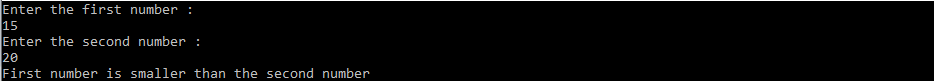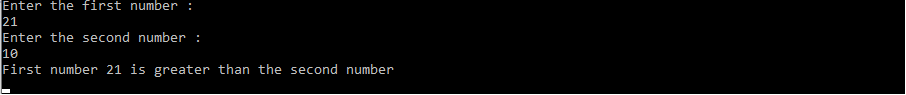# C# Program to Find Largest of Two Numbers

How to find the largest number from the given two numbers by user input – you will compare their values using either the simple if-else statement or ternary operator.

In this article, you will learn how to find the largest of two user input numbers. You will take the numbers as inputs from the user and print out a message explaining which is greater or smaller.

### Syntax using if-else

``` if (x > y)
large = x;
else
large = y;```

### Syntax using ternary operator

large = (x > y) ? x : y;

From the texts, you can understand how the ternary operator works. If x is greater than y then it will return the part after? symbol. Else, it gets the part after

C# code to find largest of two numbers using if else

You are asking for two integer numbers from user input and finding the largest one using the if-else and ternary operator.

```using System;public class Program{
public static void Main() {
int firstNumber, secondNumber;  Console.WriteLine("Enter the first number : ");
firstNumber = Convert.ToInt32(Console.ReadLine());  Console.WriteLine("Enter the second number : ");
secondNumber = Convert.ToInt32(Console.ReadLine());  if (firstNumber > secondNumber)  {
Console.WriteLine("First number is greater than the second number");
}
else  {
Console.WriteLine("First number is smaller than the second number");
}
}
}```

#### OutputYou are storing the first and the second number in firstNumber and secondNumber integer variables.

Using an if-else block, we are checking which number is the largest, and based on that we are printing one message.

### Example of ternary operator:

Similarly, you can also use a ternary operator to find the larger number and print one message based on it. The given below program explains that:

```using System;public class Program{
public static void Main() {
int firstNumber, secondNumber;
Console.WriteLine("Enter the first number : ");